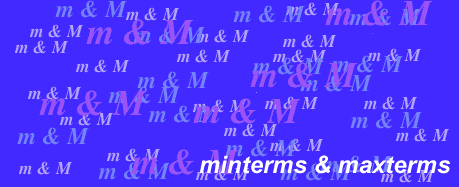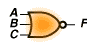This deals with the lecture subject Minterms and Maxterms. Read the specified sections in the textbook and answer the questions below. (Go back to homepage.) The truth table shown may expressed by which of the following canonical representations? ```A B C | F ---------+---- 0 0 0 | 0 0 0 1 | 0 0 1 0 | 1 0 1 1 | 0 1 0 0 | 1 1 0 1 | 0 1 1 0 | 1 1 1 1 | 0 ``` The truth table shown may be expressed by which of the following canonical expressions? ```A B C | F ---------+---- 0 0 0 | 1 0 0 1 | 0 0 1 0 | 1 0 1 1 | 1 1 0 0 | 0 1 0 1 | 0 1 1 0 | 1 1 1 1 | 0 ``` The expression AB'C' is represented by which of the following cananonical symbols? Minterm m31 must be represented by at least how many literals?Which of the following canonical expressions is generated by the NOR gate shown? Given that F = SUM m(0,1,4,5,6), which of the following correctly describes F'? Given that F = SUM m(0,2,4,6), which of the following correctly describes F'? The expression A' + B' + C is represented by which of the following canonical symbols? Given that F1 = SUM m(0,1,2) and F2 = PRD M(2,3,4,5), which of the following represent the only correct expression for F1 + F2? Given that F1 = SUM m(1,2,4,6,7) and F2 = PRD M(3,5,6) which of the following represent the only correct expression for F1*F2? (mi)' + Mi = ? Which of the following expressions is the only correct canonical representation for the function F(x,y,z) = y?